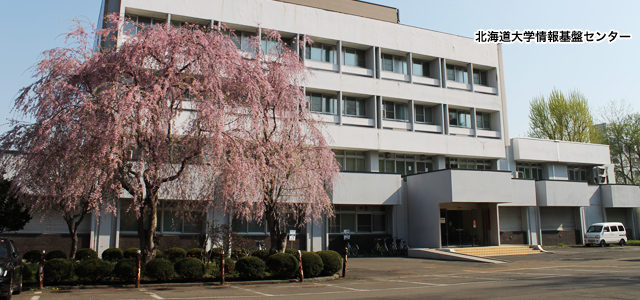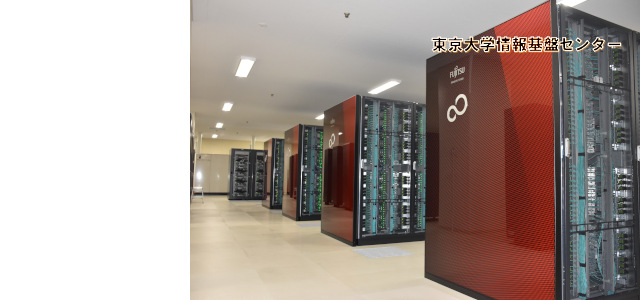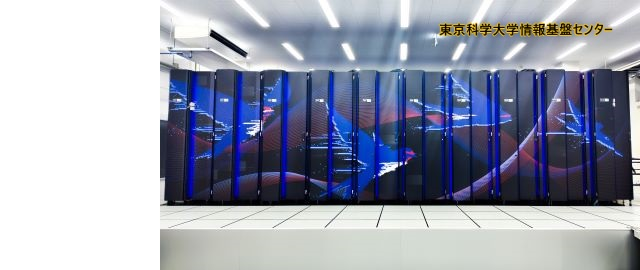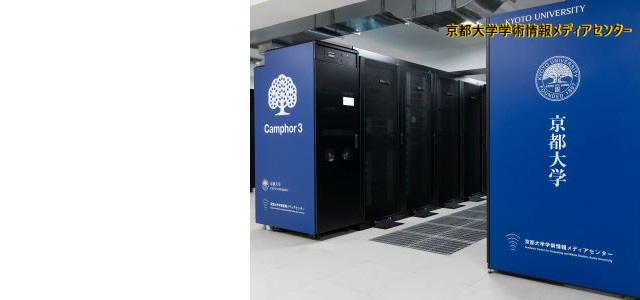•••••••••# 採択課題 【詳細】

jh210002-NAHI Developing Accuracy Assured High Performance Numerical Libraries for Eigenproblems 片桐孝洋(名古屋大学・情報基盤センター) TAKAHIRO KATAGIRI (Information Technology Center, Nagoya University) Eigenproblem is one of essential numerical problems for several numerical simulations. Its accuracy, however, is not well-assured in many conventional numerical computations. Basic Linear Algebra Subprograms (BLAS) is a frequently used to perform linear algebra computations. Ensuring the accuracy of the computational results of BLAS operations is a still crucial problem now. Even in solving linear equations using LAPACK is also a typical example, because LAPACK is rich in BLAS operations, especially matrix-matrix multiplication (MMM) operations for solving linear equations. With respect to this background, we focus on the following topics in this year based on the results of year 2: (i) Topic 1: Establishing high-performance implementation for UNC-HPC libraries, (ii) Topic 2: Developing accuracy assured libraries for real symmetric eigenproblem, (iii) Topic 3: Discussing extension to non-linear problems, and (iv) Topic 4: Prototyping and developing AT. 研究紹介ポスター ／ 最終報告書